Next: Inertia bisection Up: Geometric Based Algorithms Previous: Geometric Based Algorithms

### Coordinate bisection

The recursive coordinate bisection (RCB) algorithm  partitions the graph according to the coordinates of the vertices. For this purpose the coordinate of a vertex of the communication graph is taken as that of its corresponding node of the mesh, or that of the centroid of the corresponding element in the case of a dual graph. The RCB algorithm divides the graph into two halves according to the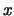-coordinates of the vertices, then further into four according to the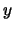-coordinates, etc.. This works well when the mesh is evenly spread over a simple domain. Otherwise it can create long boundaries with disconnected subdomains. This situation can be alleviated by the so called unbalanced recursive bisection approach , where instead of dividing the vertices into equal sets, one chooses the partitioning that minimizes the aspect ratio and divides the graph into subgraphs of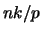and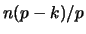vertices respectively. Here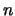is the number of vertices in the graph,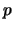the number of processors and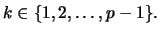By suitable choice of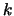at each bisection, this approach leads to subdomains of equal number of vertices but with better aspect ratios.

Figure 3 shows the result of applying the RCB to the dual graph of a mesh with 788 elements. It creates 142 inter-boundary edges.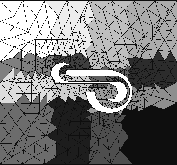Next: Inertia bisection Up: Geometric Based Algorithms Previous: Geometric Based Algorithms

2000-03-21